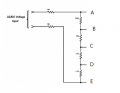# Is this circuit reasonable？

#### LowQCab

Joined Nov 6, 2012
1,372
The Question is ..........
Why do you need to change a working Circuit ?
What problem are you trying to solve ?
.
.
.

•Joined Feb 20, 2016
3,940
You could, but there is one resistor in each lead I suspect, to prevent direct connection to either probe as a safety measure.
Resistors are cheap. Use 2.

•Joined Nov 21, 2018
658
The Question is ..........
Why do you need to change a working Circuit ?
What problem are you trying to solve ?
.
.
.
Thanks.
The thing is when I check the voltage in output which can easily up to a high number say 8V when input is 25v. How can this v input to a Arduino?

Joined Nov 21, 2018
658
You could, but there is one resistor in each lead I suspect, to prevent direct connection to either probe as a safety measure.
Resistors are cheap. Use 2.
Thanks.

Joined Feb 20, 2016
3,940
•Joined Nov 21, 2018
658
That is not a real good design as, for one thing, there is no voltage limiting on the A3 input.
Look into adding clamp diodes, something like this...
View attachment 242210
"R" may be 10K, and the diodes use Schottky low forward drop types.
Out goes to A3.
Thanks.
As I know the resistance ladder can greatly reduce the input value relative to the real measuring value, if as the diagram shown put a 1 M Ohm at each end, seems there is almost no reduce ratio. Its why I am thinking or remove one 1 M, or put 2M in one side.
Not sure?

Joined Feb 20, 2016
3,940
I do not know what you mean by "almost no reduce ratio".
The ratios range from (1M + 1M) : (220K + 15K + 4.7K + 4.7K) = 2,000,000 : 244,400 = 8.183 : 1
to (1M + 1M + 220K + 15K + 4.7K) : 4.7K = 2,239,700 : 4700 = 476.5 : 1
That is, if my quick calculations are ok.

•Joined Nov 21, 2018
658
I do not know what you mean by "almost no reduce ratio".
The ratios range from (1M + 1M) : (220K + 15K + 4.7K + 4.7K) = 2,000,000 : 244,400 = 8.183 : 1
to (1M + 1M + 220K + 15K + 4.7K) : 4.7K = 2,239,700 : 4700 = 476.5 : 1
That is, if my quick calculations are ok.
Thanks.
Well, see the picture attached here, if we calculate the node "A,B,C,D,E" voltage, the 1 MOhm distributed at each end is what I mean 'almost no reduce ratio'.
lets say the node D, V = (1M + 220K + 15K + 4.7K) : (1M+4.7K), am I right?